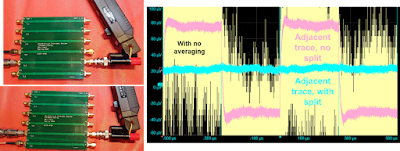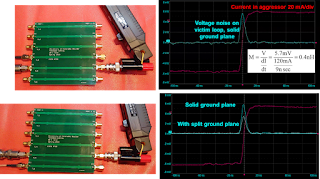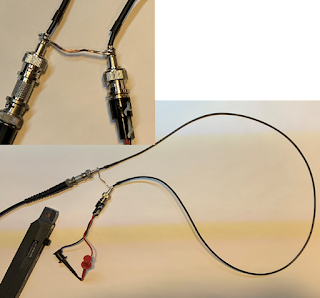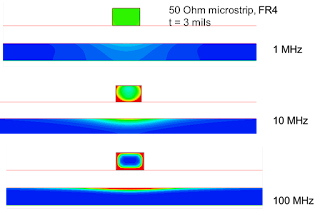## You need to test, we're here to help.

You need to test, we're here to help.

## 25 January 2023

### Eliminating DC Resistively Coupled Noise: A Signal and Power Integrity TutorialFigure 8. The measured voltage noise on the victim trace, on the other side of the ground plane gap, showing no resistively coupled cross talk on the order of 10 uV, the noise floor of the measurement.

The following is excerpted from Professor Eric Bogatin's article in the Signal Integrity Journal, The Case for Split Ground Planes. Reprinted by permission of Signal Integrity Journal.

This section continues from the discussion on Inductively Coupled Noise and Resistively Coupled Noise.

. . .

When we cut a gap in the return plane, there will be no DC current flow across the gap. There will be magnetic field coupling across the gap which is why we still see significant mutual inductance coupling between the aggressor and victim across the gap. The gap has only a small impact on this noise.

However, we would expect there would be no resistively coupled noise on the victim trace on the other side of the ground plane gap. In Figure 8, the resistively coupled noise is measured with the same scale and averaging as the noise on the victim line with no gap. The noise floor of this measurement is about 10 uV. To this level, there is no measurable resistively coupled noise, a significant reduction.

## 23 January 2023

### Inductively Coupled Noise and Resistively Coupled Noise: A Signal and Power Integrity TutorialFigure 6. Measuring the inductively coupled noise on the victim trace adjacent to the aggressor signal with no gap and separated by a gap. The inductively coupled noise is reduced by about 40% on the victim trace separated by a gap. This is a small impact.

The following is excerpted from Professor Eric Bogatin's article in the Signal Integrity Journal, The Case for Split Ground Planes. Reprinted by permission of Signal Integrity Journal.

This section continues from the discussion on Return Current at Low Frequency.

. . .

### Inductively Coupled Noise

In a plane, at frequencies below about 10 kHz, return currents will not flow under the signal path, but will spread out in the return plane. Above 10 kHz, the return currents are localized under the signal paths.

When we have two adjacent signal paths that are over a wide, continuous plane, they will show inductive cross talk at high frequency. Even with minimal overlap of the return currents, there is still loop mutual inductance between the two signal-return paths. This inductive noise is driven by the changing current, the dI/dt, in the aggressor signal-return path, which will get smaller at lower frequency.

## 18 January 2023

### Return Current at Low Frequency: A Signal and Power Integrity TutorialFigure 3. Specially configured coax cable with the front and back of the shield shorted together.

The following is excerpted from Professor Eric Bogatin's article in the Signal Integrity Journal, The Case for Split Ground Planes. Reprinted by permission of Signal Integrity Journal.

This section continues the discussion in Signal Return Paths of the equation,

Z = R + j𝛚L

Where:

Z is the loop impedance of the current loop path,

R is the series resistance of the loop and

L is the loop inductance of the path.

. . .

At low frequency, when the loop impedance is dominated by the R term, the current distribution in the return plane is NOT driven by the loop impedance, it is driven by the loop resistance. In the signal path, the current will spread out uniformly as any filament path in the signal conductor will have roughly the same resistance.

But the current filaments in the return path with the lowest R will be those which are shortest. This means that return currents will take the shortest paths, independent of the signal paths. As frequency increases, the return current will redistribute to transition from the path of lowest R to the path of lowest L.

## 16 January 2023

### Signal Return Paths: A Signal and Power Integrity TutorialFigure 1. Current distribution in the signal and return conductors at three different frequencies. The current redistribution at higher frequency is driven by the currents taking filament paths with the lowest loop inductance.
The following is excerpted from Professor Eric Bogatin's article in the Signal Integrity Journal, The Case for Split Ground Planes. Reprinted by permission of Signal Integrity Journal.

. . .

### Why Continuous Return Path Planes

The first step in engineering interconnects to reduce noise is to provide a continuous, low impedance return path to control the impedance, which controls reflection noise, and reduce the cross talk between signals that also share the same return conductor.

A wide, continuous ground plane adjacent to a signal trace will be the lowest cross talk configuration. Anything other than a wide plane means more cross talk between signal paths sharing this return conductor. This means, never add a split or gap in the return path. You would run the risk of a signal trace inadvertently crossing this discontinuity.

If a signal crosses over a split ground plane, there are two effects which compound each other. Crossing a split creates a higher impedance path for return currents that must cross the split and forces return currents from multiple signals to overlap through the same, higher impedance, common path.